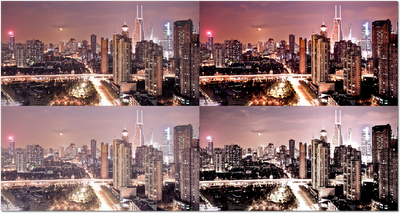🔍

10 Ocak 2012

# Levels in Renderscript

[This post is by R. Jason Sams, an Android Framework engineer who specializes in graphics, performance tuning, and software architecture. —Tim Bray]For ICS, Renderscript (RS) has been updated with several new features to simplify adding compute acceleration to your application. RS is interesting for compute acceleration when you have large buffers of data on which you need to do significant processing. In this example we will look at applying a levels/saturation operation on a bitmap.

In this case, saturation is implemented by multiplying every pixel by a color matrix Levels are typically implemented with several operations.

2. Gamma correction.

4. Clamp to the valid range.

A simple implementation of this might look like:

``````for (int i=0; i < mInPixels.length; i++) {
float r = (float)(mInPixels[i] & 0xff);
float g = (float)((mInPixels[i] >> 8) & 0xff);
float b = (float)((mInPixels[i] >> 16) & 0xff);

float tr = r * m + g * m + b * m;
float tg = r * m + g * m + b * m;
float tb = r * m + g * m + b * m;
r = tr;
g = tg;
b = tb;

if (r < 0.f) r = 0.f;
if (r > 255.f) r = 255.f;
if (g < 0.f) g = 0.f;
if (g > 255.f) g = 255.f;
if (b < 0.f) b = 0.f;
if (b > 255.f) b = 255.f;

r = (r - mInBlack) * mOverInWMinInB;
g = (g - mInBlack) * mOverInWMinInB;
b = (b - mInBlack) * mOverInWMinInB;

if (mGamma != 1.0f) {
r = (float)java.lang.Math.pow(r, mGamma);
g = (float)java.lang.Math.pow(g, mGamma);
b = (float)java.lang.Math.pow(b, mGamma);
}

r = (r * mOutWMinOutB) + mOutBlack;
g = (g * mOutWMinOutB) + mOutBlack;
b = (b * mOutWMinOutB) + mOutBlack;

if (r < 0.f) r = 0.f;
if (r > 255.f) r = 255.f;
if (g < 0.f) g = 0.f;
if (g > 255.f) g = 255.f;
if (b < 0.f) b = 0.f;
if (b > 255.f) b = 255.f;

mOutPixels[i] = ((int)r) + (((int)g) << 8) + (((int)b) << 16)
+ (mInPixels[i] & 0xff000000);
}``````

This code assumes a bitmap has been loaded and transferred to an integer array for processing. Assuming the bitmaps are already loaded, this is simple.

``````        mInPixels = new int[mBitmapIn.getHeight() * mBitmapIn.getWidth()];
mOutPixels = new int[mBitmapOut.getHeight() * mBitmapOut.getWidth()];
mBitmapIn.getPixels(mInPixels, 0, mBitmapIn.getWidth(), 0, 0,
mBitmapIn.getWidth(), mBitmapIn.getHeight());``````

Once the data is processed with the loop, putting it back into the bitmap to draw is simple.

``````        mBitmapOut.setPixels(mOutPixels, 0, mBitmapOut.getWidth(), 0, 0,
mBitmapOut.getWidth(), mBitmapOut.getHeight());``````

The full code of the application is around 232 lines when you include code to compute the constants for the filter kernel, manage the controls, and display the image. On the devices I have laying around this takes about 140-180ms to process an 800x423 image.What if that is not fast enough?

Porting the kernel of this image processing to RS (available at android-renderscript-samples) is quite simple. The pixel processing kernel above, reimplemented for RS looks like:

``````void root(const uchar4 *in, uchar4 *out, uint32_t x, uint32_t y) {
float3 pixel = convert_float4(in).rgb;
pixel = rsMatrixMultiply(&colorMat, pixel);
pixel = clamp(pixel, 0.f, 255.f);
pixel = (pixel - inBlack) * overInWMinInB;
if (gamma != 1.0f)
pixel = pow(pixel, (float3)gamma);
pixel = pixel * outWMinOutB + outBlack;
pixel = clamp(pixel, 0.f, 255.f);
out->xyz = convert_uchar3(pixel);
}``````

It takes far fewer lines of code because of the built-in support for vectors of floats, matrix operations, and format conversions. Also note that there is no loop present.

The setup code is slightly more complex because you also need to load the script.

``````        mRS = RenderScript.create(this);
mInPixelsAllocation = Allocation.createFromBitmap(mRS, mBitmapIn,
Allocation.MipmapControl.MIPMAP_NONE,
Allocation.USAGE_SCRIPT);
mOutPixelsAllocation = Allocation.createFromBitmap(mRS, mBitmapOut,
Allocation.MipmapControl.MIPMAP_NONE,
Allocation.USAGE_SCRIPT);
mScript = new ScriptC_levels(mRS, getResources(), R.raw.levels);``````

This code creates the RS context. It then uses this context to create two memory allocations to hold the RS copy of the bitmap data. Last, it loads the script to process the data.

Also in the source there are a few small blocks of code to copy the computed constants to the script when they change. Because we reflect the globals from the script this is easy.

``````        mScript.set_inBlack(mInBlack);
mScript.set_outBlack(mOutBlack);
mScript.set_inWMinInB(mInWMinInB);
mScript.set_outWMinOutB(mOutWMinOutB);
mScript.set_overInWMinInB(mOverInWMinInB);``````

Earlier we noted that there was no loop to process all the pixels. The RS code that processes the bitmap data and copies the result back looks like this:

``````        mScript.forEach_root(mInPixelsAllocation, mOutPixelsAllocation);
mOutPixelsAllocation.copyTo(mBitmapOut);``````

The first line takes the script and processes the input allocation and places the result in the output allocation. It does this by calling the natively compiled version of the script above once for each pixel in the allocation. However, unlike the dalvik implementation, the primitives will automatically launch extra threads to do the work. This, combined with the performance of native code can produce large performance gains. I’ll show the results with and without the gamma function working because it adds a lot of cost.

#### 800x423 image

DeviceDalvikRSGain
Xoom174ms39ms4.5x
Galaxy Nexus139ms30ms4.6x
Tegra 30 device136ms19ms7.2x

#### 800x423 image with gamma correction

DeviceDalvikRSGain
Xoom994ms259ms3.8x
Galaxy Nexus787ms213ms3.7x
Tegra 30 device783ms104ms7.5x

These large gains represent a large return on the simple coding investment shown above.